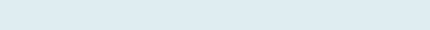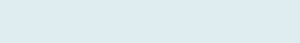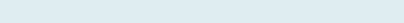# Average Based on Condition with 6 Examples

In this article, you are going to learn how to calculate AVERAGEIF and AVERAGEIFS with the help of three examples of each to find average with one more condition.

The formula illustrated below is applicable If you are looking to find Averages based on one or more conditions. Each example looks into the different cases to calculate average. If you are looking for some similar examples related to adding with criteria read this

The key examples which you will cover here are:

## Average Time between Two Dates

Let’s start, Excel AVERAGEIF Function calculates the average (arithmetic mean) of the numbers in a range that meet the applied criteria.Note: You can also evaluate the formula using a shortcut F9 function key or under the formulas, tab click on Evaluate formula.

## Average If Text Matches

To calculate Average when we have similar names in a column and averaging the Unique value of matching strings.

Query:

In this example, we have columns of Sample, Validity, and Result. Our task is to find the average of Result (Col. F) after matching the sample (Col. B).

Formula to implement:

=AVERAGEIF(\$B\$3:\$B\$23,I7,\$F\$3:\$F\$23)

Result: 3.65(3.60+3.90+3.46) = 10.96

Count= 3

(10.96/3) = 3.65

Here first it checks the criteria cell I7 i.e. “sample3” then it adds all results of column F in the given range F3 to F23 of sample3 then divides it how many times sample3 occurs.

Decrease the decimal place under the HOME tab in Excel.

Let’s start, Excel AVERAGEIF Function calculates the average (arithmetic mean) of the numbers in a range that meet the applied criteria.Note: You can also evaluate the formula using a shortcut F9 function key or under the formulas, tab click on Evaluate formula.

## Average Empty Cells Next Column

In case you want to know the average after ignoring values with text, dates, etc. comparing with other columns.

Query:

In this example, we have columns Sample, Validity, and Result. Our task is to know the average of the sample If validity is not excluded (only Empty cells) in column C.

Formula to implement:

=AVERAGEIF(D3:D23,””,F3:F23)

Result: 4.46            ‘No value with ‘Exclude’ validity

It gives average in the given range F3 to F23 on the basis of criteria “” (empty). It first adds all the numbers in Column F which are empty cells relative to column D i.e. in Validity that are without ‘exclude’ then divide by the number of occurrences (16).

If you want to know the average after ignoring values that are empty same formula will be applied only criteria change from “” (empty) to “<>” (not equal).

Formula to implement:

=AVERAGEIF(D3:D23,”<>”,F3:F23)

This formula adds all numbers in column F i.e. result from range (F3 to F23) which are with text “exclude” in validity column and then divide it with how many times exclude comes (5).

Note: : AVERAGEIF is a combination of AVERAGE and IF formula. To solve any query try to use logic in the similar manner.

## Average If More or Less than a number

To get average after including certain numbers which are more than ‘0’, without accounting for Zero or less.

Query:

In this example, we have columns Roll no., Student, and Marks. Our task is to get the average after excluding Null or Negative numbers in column (C).

Formula to implement:

=AVERAGEIF(\$D\$3:\$D\$12,”>0″)

Here it gets the average based on criteria “>0” (Greater than Zero). It first adds all of the numbers in the given range D3 to D12 of column D i.e. marks where criteria are excluding less than 0 and then divides it after counting how many times marks are greater than Zero.

Remember: Learn how you can get average after excluding error.

Note: Here we only gave range and it returns Average as the ‘Average Range’ is optional

If you want to average after excluding top and bottom 20 percent of the given data set. For this we will use TRIMMEAN formula.

Formula to implement:

=TRIMMEAN(D3:D12,20%)Average after excluding one high and one low values in the array

The first counts array (D3 to D12) i.e. 10 then it checks how much percent is given so as it excluded 20% of count (10)  i.e. 2 then it divided with 2 gives 1 so one number which is greater (50) in array will be removed and one number which is lower (-5) in the range will be removed. Then it gives the average after adding and dividing how many times it comes.

Note: With 20%, minimum of 10 data points must exist.

Now refresh our understanding with the AVERAGEIFS function before we proceed with the next three examples.

The AVERAGEIFS function in excel is a plural form of AVERAGEIF. Returns an average (arithmetic mean) number based on one or multiple conditions.

The AVERAGEIFS function has the following arguments:Now, let’s see how we can use the Excel AVERAGEIFS function on the real-time worksheet to find an average of cells that meet all of the criteria.

## Average If Similar text with Empty cells

When you want the average of similar name in multiple rows with reference to the next column where some cells are empty and some are filled with text, dates, or any non-empty info. etc.

Query:

In this example, we have columns of Employee, Sales, Amount. Our task is to know the sales average of the employee with the name Tim and not received the amount.

Formula to implement:

=AVERAGEIFS(\$C\$3:\$C\$17,\$B\$3:\$B\$17,G\$5,\$D\$3:\$D\$17,””)The formula has two criteria, first checks for Employee i.e. Tim and second, Empty cells “” in Amount (Column D) with empty cells. The Excel Average only those value in Column C that satisfy both criteria.

Note:If you want to find each Employee sales simply create a Drop-Down list.

## Average if two Criteria met in Different Column

When you want to calculate an average of numbers if both the criteria in different columns are met. In case you want an average of multiple rows after ignoring empty cells.

QUERY

In this example, we have columns Employee, Sales, and Amount. Our task is to get the average of numbers with less than 200 sales and not yet received amounts. The same can be applied for more than 200.

Formula to implement:

=AVERAGEIFS(\$C\$3:\$C\$17,\$C\$3:\$C\$17,”<200″,\$D\$3:\$D\$17,””)

The formula first checks both criteria “<200” and then “” (empty) then adds all of the numbers in the given range of column C i.e. sales (C3 to C17) If both conditions are met which are ‘less than sales 200’ and also “Blank Cells”, then return the average of those numbers.

Note: AVERAGEIFS is a combination of AVERAGE and IFS Excel functions.

## Average Time between Two Dates

When you want to know the Average of time in Multiple rows on the basis of two dates in Excel.

QUERY:

In this example, we have columns of Order Date and Total Time (Seconds). Our task is to get average of total time taken between two dates which are equals or more than Date 1 but less than or equals to Date 2.

Formula to implement:

=AVERAGEIFS(C:C,B:B,”>=”&\$F\$7,B:B,”<=”&\$F\$8)Average of time if equal to or more than date 1 and less than or equal to date 2

Result: 472.4     ‘only between two dates that include time

(Sum from C3 to C24) = 8975

(8975/19) = 472.4

First, it checks both criteria “>=”(less than or equal) and criteria  “<=”
(greater than or equal) then adds all the numbers of total time (column B) in the given range C3 to C24 which are equal or more than Date 1 and less than or equal to Date 2 then divide how many times it comes (19) so, divide it with 19 to get average.

&” operator in Excel is a type of concatenate which is used to join two strings, values or results.